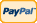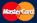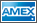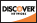Online Store Software Services News About Us My Account Español
•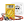I need to measure...
•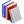I need to calculate...
•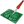Common electronic components repairs and hand soldering techniques
•Electronics, electrical engineering
•Engineering physics
•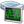Generators
•History events
•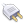Interfaces
•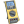Multimeters
•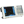Oscilloscopes
•Persona
•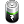Power supplies
•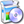Software
•Standards
•Thesaurus
•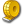Units of measurement

Filter by first letter

# Mathematical functions

Mathematical functions in digital oscilloscopes is a capability to perform mathematical operations between channels and to see the final oscillogram displayed.

Mathematical functions usually include addition, subtraction and multiplication. In most of cases division is also included. Also Fast Fourier transform (FFT) can be related to such mathematical functions. FFT allows analyzing the signals in the frequency domain (More detailed information can be found here - FFT).

The animated pictures below demonstrate the mathematical functions between the channels in a digital oscilloscope:

Addition. In the picture below which shows addition process there are three oscillograms of different colours. Colour differentiation of different signals is a very convenient method for their visualization and analysis. Such a method is widely used in Aktakom oscilloscopes. Depending on the colour scheme used the colour differentiation also can vary. In the following example: yellow oscillogram is the input signal in channel 1 (source A), blue oscillogram is the signal in channel 2 (source B) and raspberry pink (red) one is the final oscillogram. The present colour differentiation is used for all mathematical operations in Aktakom digital oscilloscopes.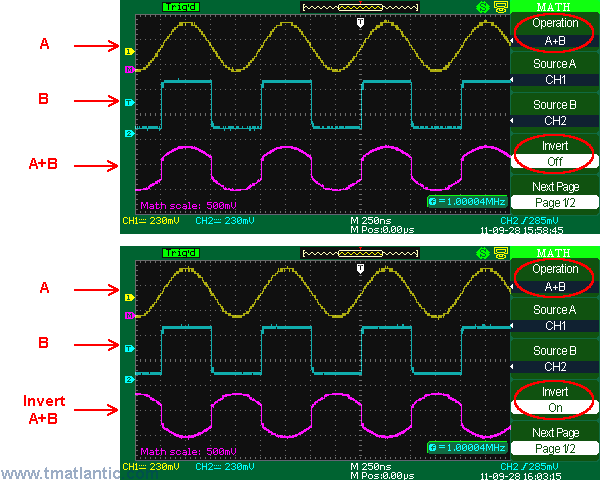Multiplication. In the picture below there is a multiplication result of channel 1 oscillogram (yellow) by channel 2 oscillogram (blue). The final oscillogram (raspberry pink (red) is located above them.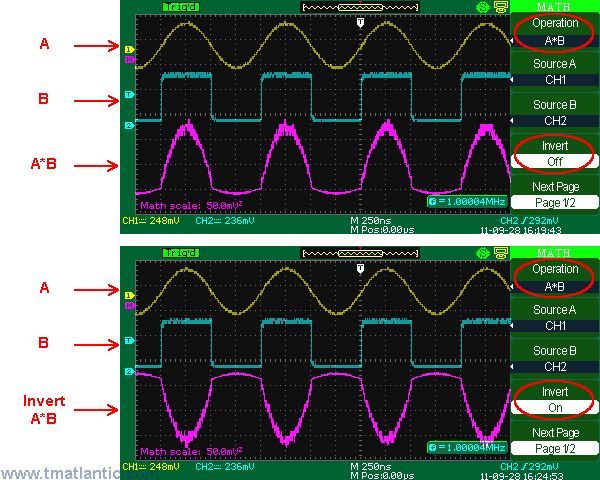Subtraction. In the picture below there is a result of channel 2 oscillogram (blue) subtracted from channel 1 oscillogram (yellow). The final oscillogram is raspberry pink (red).Division. In the picture below there is a division result of channel 1 signal (yellow oscillogram) by channel 2 signal (blue oscillogram). The final oscillogram is raspberry pink (red).The final oscillogram position for all mathematical operations as well as its vertical deflection ratio can be changed for better visualization. In addition you can use cursor manual measurement for the final oscillogram like for an ordinary signal.

Back to the list

Rating

Measurement History Events
 Yesterday Today Tomorrow
09/25/192809/25/192609/25/1820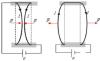Units Converter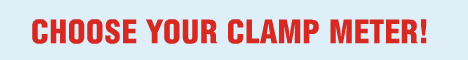Site map|Privacy policy|Terms of Use & Store Policies|How to Buy|Shipping|Payment|© T&M Atlantic, Inc., 2010-2023